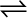7.9 Nitric oxide reacts with Br2 and gives nitrosyl bromide as per the reaction given below:

2NO (g) + Br2 (g)2NOBr (g)

When 0.087 mol of NO and 0.0437 mol of Br2 are mixed in a closed container at a constant temperature, 0.0518 mol of NOBr is obtained at equilibrium. Calculate the equilibrium amount of NO and Br2.

The given reaction is:

Now, 2 mol of NOBr are formed from 2 mol of NO. Therefore, 0.0518 mol of NOBr is formed from 0.0518 mol of NO.
Again, 2 mol of NOBr are formed from 1 mol of Br.
Therefore, 0.0518 mol of NOBr is formed from $\frac{0.0518}{2}$ mol of Br, or 0.0259 mol of NO.
The amount of NO and Br present initially is as follows:
[NO] = 0.087 mol [${\mathrm{Br}}_{2}$] = 0.0437 mol
Therefore, the amount of NO present at equilibrium is:
[NO] = 0.087 – 0.0518 = 0.0352 mol
And, the amount of Br present at equilibrium is:
[${\mathrm{Br}}_{2}$] = 0.0437 – 0.0259 = 0.0178 mol